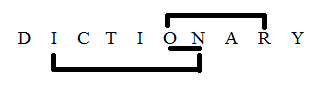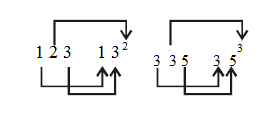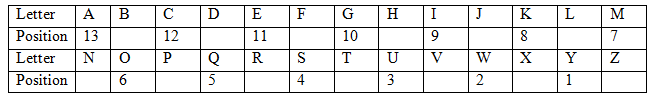# SSC CGL 2018 Practice Test Papers | Reasoning Ability (Day-32)

Dear Aspirants, Here we have given the Important SSC CGL Exam 2018 Practice Test Papers. Candidates those who are preparing for SSC CGL 2018 can practice these questions to get more confidence to Crack SSC CGL 2018 Examination.

[WpProQuiz 3187]

Click “Start Quiz” to attend these Questions and view Explanation

1) How many triangles are there in the following figure?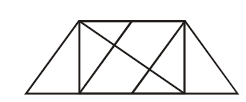a) 8

b) 16

c) 12

d) 14

2) Which of the following diagrams represents the relationships between Apple, Brinjal, Grass.

a)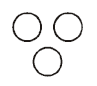b)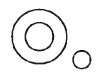c)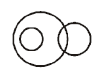d) None of these

3) Statement:

All glass is gem. No gem is diamond

Conclusion:

1. Some diamond is glass.
2. Some gem is glass.

a) Only I follow

b) Only II follows

c) Both I and II follow

d) Neither I nor II follows

4) Find the odd/ word/letters/ number from the given alternatives.

a) Mumbai

b) Kandla

c) Mysore

d) Vishakhapatham

5) Find the odd/ word/letters/ number from the given alternatives.

a) 2 : 4

b) 4 : 8

c) 6 : 18

d) 8 : 32

6) In the following questions sign ‘X’ is assigned to open state of a dice. Find the figure from the given options which can be obtained after closing the figure (X).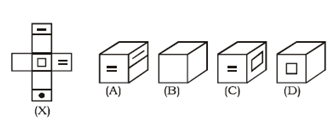a) Only A
b) Only A, C and D

c) Only B

d) None of these

7) How many pairs of letters are there in the word “DICTIONARY” which has as many letters between them in the words as they have between them in the English alphabet?

a) One

b) Two

c) Three

d) More than three

8) Select the related word/letters/number from the given alternatives.

123 : 132: : 335 : ?

a) 232

b) 353

c) 253

d) 252

9)  If all the alphabets at even places are left out, then remaining alphabets are numbered 1, 2, 3….. from right side then what is the position of I from right end?

a) 11

b) 13

c) 6

d) 9

10) Arrange the words as per the order in dictionary.

i) Mountain

ii) Mould

iii) Micron

iv) Mushroom

a) iii, ii, i, iv

b) I, ii, iii, iv

c) iv, ii, ii, i

d) I, iv, iii, ii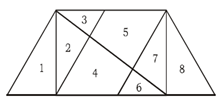Required. Triangles = 1, 2, 3, 6, 7, 8, (2, 3), (7, 6), (3, 5), (4, 6), (2, 4, 6), (3, 5, 7), (6, 7, 8) and (1, 2, 4, 6)

Apple is a fruit

Brinjal is avvegetable

Grass is a herb.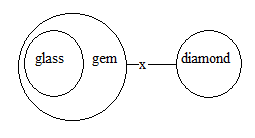Except option (C), all are harbor cities.

4^2/2=16/2=8

6^2/2=36/2=18

8^2/2=64/2=32